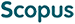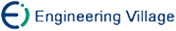陈辉焱, 王连强, 吕述望. 关于HFE密码系统的密钥问题研究[J]. 计算机研究与发展, 2007, 44(7): 1205-1210.
 引用本文: 陈辉焱, 王连强, 吕述望. 关于HFE密码系统的密钥问题研究[J]. 计算机研究与发展, 2007, 44(7): 1205-1210.Chen Huiyan, Wang Lianqiang, Lü Shuwang. A Study of Key Problems of HFE Cryptosystem[J]. Journal of Computer Research and Development, 2007, 44(7): 1205-1210.
 Citation: Chen Huiyan, Wang Lianqiang, Lü Shuwang. A Study of Key Problems of HFE Cryptosystem[J]. Journal of Computer Research and Development, 2007, 44(7): 1205-1210.## A Study of Key Problems of HFE Cryptosystem

• 摘要: 多变量密码系统的设计与分析在理论探索与实际应用中扮演着重要的角色.在现有的多变量密码系统中，由Partarin在1996年提出的HFE密码系统被认为是最有前途的.在许多应用中，因HFE密码系统快速的公钥加密等原因，它还是一个很有前途的公钥密码.它的安全性是基于有限域F上的多元二次方程组的求解问题.在HFE公钥密码系统中，密钥问题是一个很重要的问题，但目前还没有文献给出这方面的详细研究.给出了非平凡公钥和非平凡私钥的概念，可以证明在一个给定可逆线性映射φ:K→Fn(其中K是F的n次扩展，F的特征为2)及其逆的作用下，对每一个非平凡公钥都有qn(n+1)∏ni=1(q\+i-1)\+2个非平凡私钥与之相对应，同时得出结论：求有限域F上的任意二次方程组的解(方程的个数m小于等于变元的个数n)都可转化求有限域K(K是有限域F的n次扩展)上多项式方程的根.另外还给出了有限域F上HFE密码系统的两类弱密钥.这些结果不但能加深对HFE密码系统的理解，而且也可能会产生对HFE密码系统的新的攻击.

Abstract: The design and analysis of multivariate cryptosystems play an important role in theory research and practical use. The HFE cryptosystem presented by Jacques Patarin in 1996 has long been regarded as the most promising one of multivariate cryptosystems, and is a promising public key cryptosystem with many practical applications: very fast or very short digital signatures, fast public key encryption, etc. The security of the HFE cryptosystem is based on the problem of solving a system of multivariate quadratic equations over a finite field F. The problems about keys, which haven't been investigated in detail in literature, are very important in the HFE cryptosystem. Nontrivial public key and nontrivial private key are defined. If a reversible linear mapping φ:K→Fn is given where K is an extension of degree n of the finite field F and char(F)=2, there are the corresponding qn(n+1)∏ni=1(q\+i-1)\+2 nontrivial private keys for per nontrivial public key. A conclusion that solving a system of m multivariate quadratic equations with n variants (m≤n) over F is reduced to finding root of polynomial equation over K. This result leads to a deeper understanding of HFE and may yield a new kind of attack. In addition, two categories of weak keys on the HFE cryptosystem over F are introduced./下载:  全尺寸图片 幻灯片
• 分享
• 用微信扫码二维码

分享至好友和朋友圈